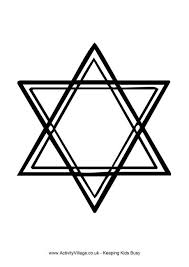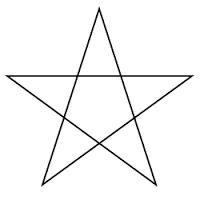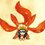# Area of hexagon through a starWe can find area of a regular hexagon by creating a star with two equilateral triangles. Let the side of them be 3 cm. Add their area and subtract from it the area of 6 small triangles. Divide by 2 to get the area of the regular hexagon of length 1 cm.

Here area of big triangles=2X(9/4X3^(1/2))
area of small triangles=6X(1/4X3^(1/2))
area of hexagon= (9X3^(1/2)/2-3X3^(1/2)/2)/2= 6X3^(1/2)/4=3X3^(1/2)/2 Also, can someone find area of a pentagon through a pentagonal starHint: Consider each small line segment of the star to be 1Note by Prince Loomba
5 years, 3 months ago

This discussion board is a place to discuss our Daily Challenges and the math and science related to those challenges. Explanations are more than just a solution — they should explain the steps and thinking strategies that you used to obtain the solution. Comments should further the discussion of math and science.

When posting on Brilliant:

• Use the emojis to react to an explanation, whether you're congratulating a job well done , or just really confused .
• Ask specific questions about the challenge or the steps in somebody's explanation. Well-posed questions can add a lot to the discussion, but posting "I don't understand!" doesn't help anyone.
• Try to contribute something new to the discussion, whether it is an extension, generalization or other idea related to the challenge.

MarkdownAppears as
*italics* or _italics_ italics
**bold** or __bold__ bold
- bulleted- list
• bulleted
• list
1. numbered2. list
1. numbered
2. list
Note: you must add a full line of space before and after lists for them to show up correctly
paragraph 1paragraph 2

paragraph 1

paragraph 2

[example link](https://brilliant.org)example link
> This is a quote
This is a quote
    # I indented these lines
# 4 spaces, and now they show
# up as a code block.

print "hello world"
# I indented these lines
# 4 spaces, and now they show
# up as a code block.

print "hello world"
MathAppears as
Remember to wrap math in $$ ... $$ or $ ... $ to ensure proper formatting.
2 \times 3 $2 \times 3$
2^{34} $2^{34}$
a_{i-1} $a_{i-1}$
\frac{2}{3} $\frac{2}{3}$
\sqrt{2} $\sqrt{2}$
\sum_{i=1}^3 $\sum_{i=1}^3$
\sin \theta $\sin \theta$
\boxed{123} $\boxed{123}$

Sort by:

Well, the angles of the smaller triangle are $\frac{2\pi}{5}, \frac{2\pi}{5}, \frac{\pi}{5}$.
You would need to derive the area of such a triangle, and then use the exact same argument that you made.

- 5 years, 3 months ago

I used herons formula for (5 big triangles of side (2,2,3)-2 X (area of 5 small triangles(1,1,1)))/5 but the answer is wrong

- 5 years, 3 months ago

If there is a regular polygon of n sides($n\geq 3)$,then its interior angle is equal to ($\frac{2n-4}{n}\times 90).$Now substitute $n=5.$
You get each interior angle to be equal to $108^\circ.$
Now take $\triangle ABC,$
Using Cosine Rule,
$k^2=a^2+b^2-2bc.cosB\Rightarrow k^2=1+1-2.cos108^\circ\Rightarrow k=\sqrt{2.62}\Rightarrow k=1.62$ $units.$
By using heron's formula,we get the area of $\triangle ABC=0.47$ ${units}^2.\triangle AED$ has the area as that of \triangle ABC since both triangles are congruent.So,the length of AD is $1.62$ $units.$
Now take $\triangle ACD,$
Using heron's formula,we get the area of $\triangle ACD=0.77$ ${units}^2.$
Therefore,the area of the pentagon ABCDE$=$area of $\triangle ABC+$area of $\triangle AED+$area of $\triangle ACD$ $=0.47+0.47+0.77$
$=\boxed{1.71 {units}^2}.$

- 5 years, 2 months ago

But I asked to calculate area through a star

- 5 years, 2 months ago

i know it bro!!

- 5 years, 2 months ago

But if people read this then they may find it correct

- 5 years, 2 months ago

okkkk!!!

- 5 years, 2 months ago